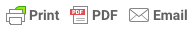# What is a hypothesis?

## What is a hypothesis?

By Saul McLeod, updated August 10, 2018

A hypothesis (plural hypotheses) is a precise, testable statement of what the researcher(s) predict will be the outcome of the study.

This usually involves proposing a possible relationship between two variables: the independent variable (what the researcher changes) and the dependent variable (what the research measures).

In research, there is a convention that the hypothesis is written in two forms, the null hypothesis, and the alternative hypothesis (called the experimental hypothesis when the method of investigation is an experiment).

### Alternative Hypothesis

The alternative hypothesis states that there is a relationship between the two variables being studied (one variable has an effect on the other).

It states that the results are not due to chance and that they are significant in terms of supporting the theory being investigated.

### Null Hypothesis

The null hypothesis states that there is no relationship between the two variables being studied (one variable does not affect the other).

It states results are due to chance and are not significant in terms of supporting the idea being investigated.

### Nondirectional Hypothesis

A two-tailed non-directional hypothesis predicts that the independent variable will have an effect on the dependent variable, but the direction of the effect is not specified.

E.g., there will be a difference in how many numbers are correctly recalled by children and adults.

### Directional Hypothesis

A one-tailed directional hypothesis predicts the nature of the effect of the independent variable on the dependent variable.

E.g., adults will correctly recall more words than children.

#### Can a hypothesis be proven?

Upon analysis of the results, an alternative hypothesis can be rejected or supported, but it can never be proven to be correct.

We must avoid any reference to results proving a theory as this implies 100% certainty, and there is always a chance that evidence may exist which could refute a theory.

#### How to Write a Hypothesis

• 1. To write the alternative and null hypotheses for an investigation, you need to identify the key variables in the study.

The independent variable is manipulated by the researcher and the dependent variable is the outcome which is measured.

• 2. Operationalized the variables being investigated.

Operational variables (or operationalizing definitions) refer to how you will define and measure a specific variable as it is used in your study.

• 3. Decide on a direction for your prediction. If there is evidence in the literature to support a specific effect on the independent variable on the dependent variable, write a directional (one-tailed) hypothesis.

If there are limited or ambiguous findings in the literature regarding the effect of the independent variable on the dependent variable, write a non-directional (two-tailed) hypothesis.
• 4. Write your hypothesis. A good hypothesis is short (i.e. concise) and comprises clear and simple language.

#### What Are Examples of a Hypothesis?

Let’s consider a hypothesis that many teachers might subscribe to: that students work better on Monday morning than they do on a Friday afternoon (IV=Day, DV=Standard of work).

Now, if we decide to study this by giving the same group of students a lesson on a Monday morning and on a Friday afternoon and then measuring their immediate recall on the material covered in each session we would end up with the following:

• The alternative hypothesis states that students will recall significantly more information on a Monday morning than on a Friday afternoon.
• The null hypothesis states that there will be no significant difference in the amount recalled on a Monday morning compared to a Friday afternoon. Any difference will be due to chance or confounding factors.

The null hypothesis is, therefore, the opposite of the alternative hypothesis in that it states that there will be no change in behavior.

At this point, you might be asking why we seem so interested in the null hypothesis. Surely the alternative (or experimental) hypothesis is more important?

Well, yes it is. However, we can never 100% prove the alternative hypothesis. What we do instead is see if we can disprove, or reject, the null hypothesis.

If we reject the null hypothesis, this doesn’t really mean that our alternative hypothesis is correct – but it does provide support for the alternative / experimental hypothesis.